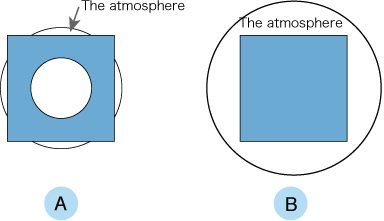ページ内のクイックリンク
HOME  >  english  >  Cubic Earth  >  Where is the atomosphere ?

Cubic Earth

# Where is the atomosphere ?

## How much does the atmosphere extend?

The atmosphere that covers the real Earth contains nitrogen (80%) and oxygen (20%). We cannot feel the weight of the atmosphere, but it has a weight. used in weather forecasts is produced by the weight of the atmosphere.

How do you think the atmospheric pressure on the cubic Earth?

On the real Earth, the atmospheric pressure at the sea level is almost 1013hPa anywhere on the Earth. This means that we can travel anywhere on the Earth without help of the space suit.

We cannot feel how much atmosphere is piled on the surface of the real Earth, because we are inside of . However, we know that the atmospheric pressure decreases, when we climb a mountain, because the amount of the atmosphere piled above is reduced on the mountain. The atmospheric pressure at the altitude of 100 km is 1/ 1,000,000 of the value at sea level. Scientists consider that this altitude is the boundary between the atmosphere and the space, i.e., the thickness of the atmosphere is approximately 100 km.

## Let us calculate the atmospheric pressure at the sea level.

We cannot weigh the weight of the atmosphere like we weigh weights of meat and vegetables. However, we know that the weight of the atmosphere on a ground of 1cm2 is the same with that of the mercury of 76 cm high. The density of the mercury is 13.6 g/cm3. Therefore, the mercury of 76 cm high with the cross section of 1 cm2 is:

13.6 g/cm3 × 76 cm ＝ 1033.6 g/cm2

This means that approximately 1 kg of the air is piled on a ground with 1cm2 at sea level. Let M the total mass of the atmosphere that covers the real Earth. Then,

M ＝ 5.1×1018 kg

Let us imagine the atmosphere on the cubic Earth. We assume that the equal amount of the air is distributed on the 6 sides of the cube. Then, the mass of the atmosphere piled on each side is

M/6 ＝ 0.85×1018 kgFig. 2 Outer appearance of the atmospheres around the cubic earth.

Even on the cubic Earth, the atmosphere is piled in a form of the sphere, because the gravity attracts the air toward the center of the cube. When the amount of the air is not enough, the air is piled like a convex lens as shown in Fig. 2(a). When the amount of the air is enough, the cube is covered by the air as shown in Fig. 2(b).

このページのトップへ